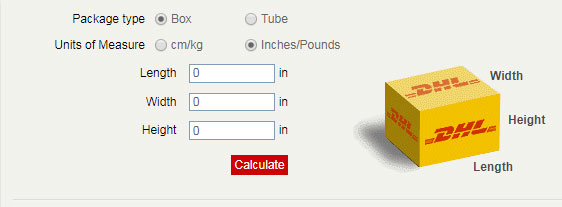# Volumetric Weight Calculation Methods For DHL FedEx UPS TNT express

International shipping cost depends on actual weight and Volumetric Weight. Big volume, light weight these cargo such as Empty plastic bottle / Sofa / Chair... maybe have Volumetric Weight. Volumetric Weight is also named dimensional weight.

Why use volumetric Weight for calculating the shipping cost? It is very simple to understand: the light cargo occupied space more than the heavy cargo.

Different international shipping ways have different volumetric weight methods. Here are all shipping way volumetric weight computing methods.

## Volumetric Weight Calculation Method For Express

Use DHL express, FedEx express, UPS courier the volumetric weight computing methods as bellow:

 CM/KG; Inches/Pounds; length*width*height/5000; length*width*height/139;

Check each box size and use over methods you can get the volumetric weight. For example, a carton the three size are : 50 CM(L)* 68CM (W)*38CM(H), the volumetric weight= (50*68*38)/5000=25.84KG=26KG. If this carton actual weight is 5 KG, shipping from China to US by FedEx IP cost is RMB 38/KG. The total FedEx cost is RMB38/KG*26KG=980 RMB. It is not RMB38/KG*5KG=190RMB.

One shipment is more than 1 cartons DHL / FedEx /TNT the total volumetric weight are each carton's weight added together. For example. 6 cartons each size is 38CM*18CM*58CM by DHL express from China to Canada, total volumetric weight is 6(38*18*58)/5000=47.6KG=48KG.

### FedEe Volumetric Weight calculation methods

• Measure the length*/ width and height of the consignment in centimeters.
• Calculate the volume and divide it by 5,000 to get the dimensional weight in kilograms.
• If the dimensional weight is greater than the consignment’s actual weight, use the dimensional weight to calculate the FedEX rate.

Volumetric WEIGHT FACTOR = (L X W X H) in cms/5000 = Kilograms

Note: Length is defined as the longest side of the packages.

### UPS Express Volumetric Weight calculation methods

UPS express volumetric weight is different rule. UPS express not use total each volumetric weight adding together, it depends on one Each carton that maybe include volumetric weight, volumetric weight and actual weight in one package which bigger use which weight. For example: There are two packages.

 Package Dimensions/Package GrossWeight(KGS) DHLvolumetricweight(KG) UPSvolumetricweight(KG) UPSrateWeight(KG) 1 50*28*58cm 8.20 16.24 17 17 1 26*38*48cm 6.50 9.48 10 10 1 8*18*28CM 16.00 0.8 1 16 Total: 3 30.70 26.52 28 43

Over this packing list show the different chargeable weight calculation methods for DHL/FedEx/TNT and UPS. If there is volumetric weight use UPS express the charges weight are more than other international express company.## Volumetric Weight Calculation for international air freight

Air freight volumetric weight Calculation method is (length*width*height/6000)

## Volumetric Weight Calculation for LCL sea freight

How to calculate volumetric weight for road freight

volumetric weight vs actual weight